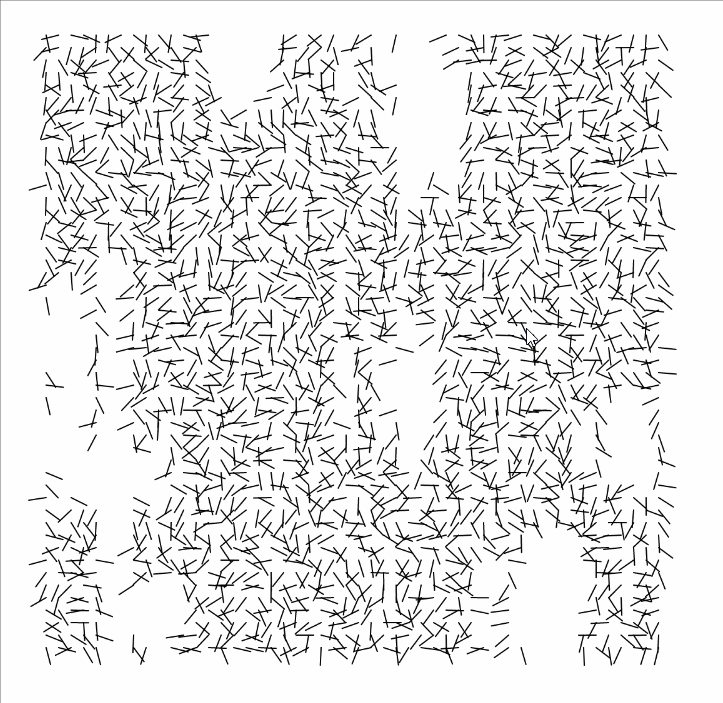# sheep-Interruptions

1. A square canvas
2. Lines of equal size
3. Lines are scattered in various different directions.
4. Lines don’t always intersect with each other
5. In certain places less lines are drawn
6. Majority of lines face one direction for each piece
7. Around 55 lines on bottom and top
8. There are around 55 lines on the left and right sides
9. Spaces exist where no lines are drawn, essentially skipped over
10. These spaces can be large, other times they are smallI am so much more appreciative of the algorithm Vera made. It seems as if every time I thought I had a pattern I liked there was another version that looked horrible. In the end, I don’t think I got to Vera’s level of consideration. In the end, Vera’s interruptions feel like the lines around the interruptions are affected by the gaps. If the lines are going perpendicular to the gaps, they stop. Sometimes the lines dip into the interruptions- almost as if they have physical properties. Her distributions feel much more natural, which I appreciated considering she didn’t use Perlin noise. I’m kind of happy with how it came out but it really doesn’t feel like an accurate representation.

```1 2 3 4 5 6 7 8 9 10 11 12 13 14 15 16 17 18 19 20 21 22 23 24 25 26 27 28 29 30 31 32 33 34 35 36 37 38 39 40 41 42 43 44 45 46 47 48 49 50 51 52 53 54 55 56 57 58 59 60 61 62 63 64 65 66 67 68 69 70 71 72 73 74 75 76 77 78 79 80 81 82 83 84 85 var state = true;   function setup() { createCanvas(600, 600);     } //Paul Bourkes function draw() { if (state === true) { var angle; var lineLength = 17; var lines = []; var numLines = 50; var borders = 50; var distance = 10; var rand = []; var radiiX = []; var radiiY = []; var xoff = 0.0; var xCoords = []; var yCoords = [];     var rand1 = round(random(1));   for (var x = 0; x < 10; x++) {   append(xCoords, round(random(0, 50))); } for (var y = 0; y < 10; y++) {   append(yCoords, round(random(0, 50))); } for (var i = 0; i < 10; i++) { append(rand, round(random(0, 50))); }     background(255); for (var l = 0; l < numLines; l++) { append(radiiX, 1 + random(5)); append(radiiY, 1 + random(6));   } for (var a = 0; a < numLines; a++) {   for (var b = 0; b < numLines; b++) { var skip = false; angle = random(1) * PI;   var radiusX = radiiX[a]; var radiusY = radiiY[a]; if (rand1 == 0) { for (var u = 0; u < xCoords.length; u++) { if ((a + radiusX > xCoords[u] && a - radiusX < xCoords[u] && b + radiusY > yCoords[u] && b - radiusY < yCoords[u])) { skip = true; } } if (skip == false) { line(a * distance+borders, b * distance+borders, (a * distance+borders) + (cos(angle) * lineLength), (b * distance+borders)+sin(angle)*lineLength); } } if (rand1 == 1) { for (var u = 0; u < xCoords.length; u++) { if ((a + radiusX > xCoords[u] && a - radiusX < xCoords[u] && b + radiusY > yCoords[u] && b - radiusY < yCoords[u])) { skip = true; } } if (skip == false) { line(a * distance+borders, b * distance+borders, (a * distance+borders) + (cos(angle) * lineLength), (b * distance+borders) +sin(angle)*lineLength); }   } } } } state = false; }       function mouseClicked() { state = true; }```